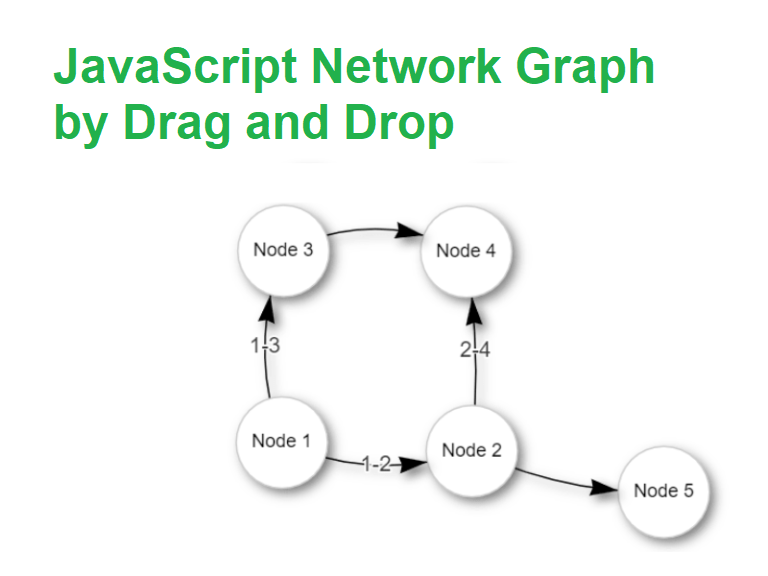# Vis Js Network Save Position – Create Network Graph JavaScriptWe will see use of vis js network save position in this blog.

I needed to create a Network graph with lots of nodes and edges and it was becoming very hard, as due to lots of edges, it was becoming chaotic.

I was using vis.js, my favorite for network graphs. But it was not generating the graph I needed. I tried a lots of layouts, nodes, edges, and physics options. I spent a whole day tweaking settings, but due to large number of nodes, I was not getting a satisfactory graph.

Now, vis.js lets us drag and change the positions of nodes. But, I was unaware of the fact that once we have dragged all the nodes and get required positions, we could save that positions and load whenever needed.

I was looking over stackoverflow for maintaining big graphs using vis.js and I came across this stackoverflow answer by totymedli.

That helped me to save network position with vis js.

So, we can use storePositions() to load the X and Y coordinates to your dataset. We can save them, then just expand the nodes with the saved coordinates when we initialize the network later.

Suppose we have a vis.js graph.

Easiest way for JavaScript Network Graph Visualization – Vis.js

So, we can drag the nodes and make the layout of graph as we wanted it to be.

Then we can use storePositions.

``network.storePositions()``

This is available at network.data.nodes.

``const nodePositions = data.nodes.map(({ id, x, y }) => ({ id, x, y }))``

Its saved in nodePositions variable. So, next time we load the graph, we can update graph layout using this variable.

``copy(nodePositions)``

This would copy the value in this variable, which is just the array of coordinates of positions. So, we can save it somewhere.

And, next we can load it and use it to update the graph.

``nodePositions.forEach(nodePosition => data.nodes.update(nodePosition))``

## Update: Vis Js Network Save Positions

So, later I also had to use dynamic filter in vis.js. And using DataView for dynamic filter, the storePositions was no longer working. storePositions was giving error.

Uncaught TypeError: Cannot read property ‘x’ of undefined

In a discussion, I found out storePositions would not work with dataview.

So, the alternative here is to use getPosition and later put them in the dataset.

However the data returned by it (getPosition) is incompatible for data.update(). So, changing a bit of structure.

``````let theNodePosition = []
let theEdgePosition = []

function savePosition() {
let gpPositions = network.getPositions()
let edgePositions = network.body.edges;

// Either way
for (const [key, value] of Object.entries(gpPositions)) {
let tempObj = {
id: parseInt(key),
x: value.x,
y: value.y
}
theNodePosition.push(tempObj)
}
for(let edgeId in edgePositions){
theEdgePosition.push({ from:edgePositions[edgeId].from['id'], to: edgePositions[edgeId].to['id'] });
}
}``````

``````theNodePositions.forEach(nodePosition => nodes.update(nodePosition))